Gauge your potential with Aakash BYJU'S JEE Main'23 Mock Test Gauge your potential with Aakash BYJU'S JEE Main'23 Mock Test

# JEE Main 2021 March 18 – Shift 2 Chemistry Question Paper with Solutions

JEE aspirants can find the solutions of JEE Main 2021 March 18 Shift 2 Chemistry paper on this page. Students are recommended to revise these solutions so that they can be more familiar with the type of questions asked for the JEE Main exam. These solutions are designed by expert teachers at BYJU’S. Learning these solutions will help students to improve their speed and accuracy. Students can download the solutions in PDF format also.

### JEE Main 2021 March 18th Shift 2 Chemistry Question Paper

SECTION-A

Question 1. Consider the below-given reaction, the product ‘X’ and ‘Y’ respectively are: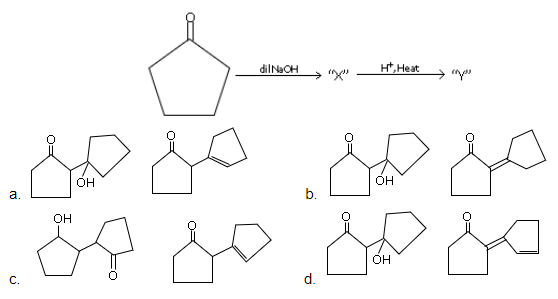Solution: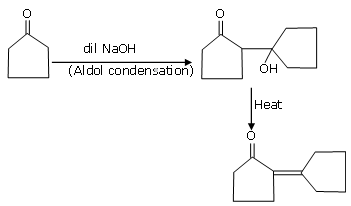Question 2. The charges on the colloidal CdS sol. and TiO2 sol. are, respectively

1. a. positive and negative
2. b. negative and negative
3. c. negative and positive
4. d. positive and positive

Solution:

CdS → Sulphide sol. → Negative sol.

Question 3. The oxide that shows a magnetic property is:

1. a. SiO2
2. b. Na2O
3. c. Mn3O4
4. d. MgO

Solution:

Mn3O4 is paramagnetic due to the presence of unpaired electrons.

Question 4. Given below are two statements:

Statement I: Bohr’s theory accounts for the stability and line spectrum of Li+ ion.

Statement II: Bohr’s theory was unable to explain the splitting of spectral lines in the presence of a magnetic field.

In the light of the above statements, choose the most appropriate answer from the options given below:

1. a. Both statement I and statement II are true
2. b. Statement I is true but statement II is false
3. c. Statement I is false but statement II is true
4. d. Both statement I and statement II are false

Solution:

S-1 → false

S-2 → True

Hence, option c is correct.

Question 5. Match List-I with List-II:

 List-I List-II (a) Mercury (i) Vapour phase refining (b) Copper (ii) Distillation Refining (c) Silicon (iii) Electrolytic Refining (d) Nickel (iv) Zone Refining

Choose the most appropriate answer from the option given below:

1. a. (a)-(ii), (b)-(iii), (c)-(i), (d)-(iv)
2. b. (a)-(ii), (b)-(iv), (c)-(iii),(d)-(ii)
3. c. (a)-(i), (b)-(iv), (c)-(ii), (d)-(iii)
4. d. (a)-(ii), (b)-(iii), (c)-(iv), (d)-(i)

Solution:

(a) The pyrometallurgical extraction of mercury from its ore is essentially a distillation process

(b) The purification of metals by electrolysis. It is commonly applied to copper. A large piece of impure copper is used as the anode with a thin strip of pure copper as the cathode

(c) Zone Refining. Zone refining is a very useful method to get metals with very high purity such as silicon and germanium. It is also referred to as zone melting

(d) Mond's process for extraction of nickel and Van-Arkel Method for preparing ultra-pure titanium are based on the principle of vapour phase refining.

Question 6. Match List-I with List-II:

 List-I List-II (Class of Chemicals) (Example) (a) Antifertility drug (i) Meprobamate (b) Antibiotic (ii) Alitame (c) Tranquilizer (iii) Norethindrone (d) Artificial Sweetener (iv) Salvarsan

Choose the most appropriate answer from the option given below:

1. a. (a)-(iv), (b)-(iii), (c)-(ii), (d)-(i)
2. b. (a)-(ii), (b)-(iii), (c)-(iv), (d)-(i)
3. c. (a)-(ii), (b)-(iv), (c)-(i), (d)-(iii)
4. d. (a)-(iii), (b)-(iv), (c)-(i), (d)-(ii)

Solution:

(a) Antifertility drug → Norethindrone

(b) Antibiotic → Salvarsan

(c) Tranquilizer → Meprobamate

(d) Artificial sweetener → Alitame

Question 7. Main Products formed during a reaction of 1-methoxy naphthalene with hydroiodic acid are: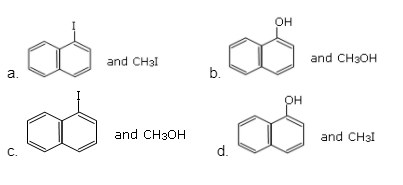Solution: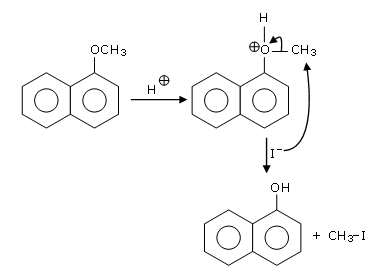Question 8. Consider the given reaction, percentage yield of: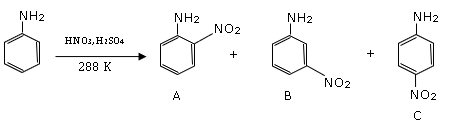1. a. A > C > B
2. b. B > C > A
3. c. C > B > A
4. d. C > A > B

Solution: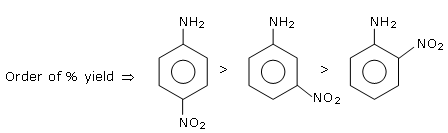Question 9. An organic compound “A” on treatment with benzene sulphonyl chloride gives compound B. B is soluble in dil. NaOH solution. Compound A is:

1. a. C6H5–N–(CH3)2,
2. b. C6H5–NHCH2CH3
3. c.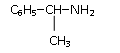4. d. C6H5–CH2 NH CH3

Solution: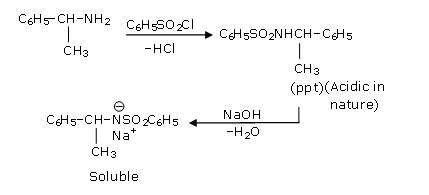Question 10. The first ionization energy of magnesium is smaller as a compound to that of elements X and Y but higher than that of Z. The elements X, Y and Z, respectively are:

1. a. argon, lithium and sodium
2. b. chlorine, lithium and sodium
3. c. neon, sodium and chlorine
4. d. argon, chlorine and sodium

Solution:

Order of I.E.

3rd period → Na < Al < Mg < Si < S < P < Cl < Ar

Question 11. In the following molecule: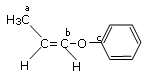The hybridisation of Carbon a, b and c respectively are:

1. a. sp3, sp2, sp2
2. b. sp3, sp2, sp
3. c. sp3, sp, sp
4. d. sp3, sp, sp2

Solution:

a → sp3

b → sp2

c → sp2

Question 12. In the reaction of hypobromite with amide, the carbonyl carbon is lost as:

1. a. HCO3
2. b. CO32–
3. c. CO2
4. d. CO

Solution:

CO32–

Question 13. The oxidation states of nitrogen in NO, NO2, N2O and NO3 are in the order of

1. a. NO2 > NO3 > NO > N2O
2. b. N2O > NO2 > NO > NO3
3. c. NO3 > NO2 > NO > N2O
4. d. NO > NO2 > NO3> N2O

Solution:

O.S. of 'N'

NO → +2

NO2 → +4

N2O → +1

NO3 → +5

Decreasing order of ox. state of 'N' is as follows

NO3 > NO2 > NO > N2O

Question 14. Match List-I and List-II:

 List-I List-II (a) Be (i) treatment of cancer (b) Mg (ii) extraction of metals (c) Ca (iii) incendiary bombs and signals (d) Ra (iv) windows of X-ray tubes - (v) bearings for motor engines

Choose the most appropriate answer from the option given below:

1. a. (a)-(iii), (b)-(iv), (c)-(ii), (d)-(v)
2. b. (a)-(iv), (b)-(iii), (c)-(i), (d)-(ii)
3. c. (a)-(iv), (b)-(iii), (c)-(ii), (d)-(i)
4. d. (a)-(iii), (b)-(iv), (c)-(v), (d)-(ii)

Solution:

Fact (NCERT)

(a) Be is used in bearings of motor engines. The higher strength copper-beryllium compared to alternative bronze landing gear materials allows the bearings to be made smaller and lighter.

(b) When combined with water while burning it releases gas and oxygen. Magnesium is used in either powdered or solid form as an incendiary agent for both illumination and antipersonnel purposes.

(c) Pure calcium metal is used as a reducing agent in the preparation of other types of metal, such as thorium and uranium and zirconium.

(d) Due to its radioactive nature Ra- is used in the treatment of cancer.

Question 15. Deficiency of vitamin K causes:

1. a. Cheilosis
2. b. Increase in blood clotting time
3. c. Increase in the fragility of RBCs
4. d. Decrease in blood clotting time

Solution:

The deficiency of vitamin "K" causes an increase in blood clotting time.

Question 16. Given below are two statements:

Statement I: C2H5OH and AgCN both can generate nucleophiles.

Statement II: KCN and AgCN both will generate nitrile nucleophiles with all reaction conditions.

Choose the most appropriate option:

1. a. Statement I is false but statement II is true
2. b. Statement I is true but statement II is false
3. c. Both statement I and statement II are false
4. d. Both statement I and statement II are true

Solution:

Þ C2H5OH & AgCN both can generate nucleophiles.

Þ AgCN & KCN both do not generate nitrite nucleophiles in all reaction conditions.

Question 17. Given below are two statements:

Statement I: Non-biodegradable wastes are generated by thermal power plants.

In the light of the above statements, choose the most appropriate answer from the options given below.

1. a. Statement I is false but statement II is true
2. b. Statement I is true but statement II is false
3. c. Both statement I and statement II are false
4. d. Both statement I and statement II are true

Solution:

Fact (NCERT-Based)

Statement II: Biodegradable detergents do not contain phosphate salts as these type of salts lead to over enrichment of phosphate causing water bodies to be choked with algae and other plants (eutrophication). So statement II is false.

Question 18. A hard substance melts at high temperature and is an insulator in both solid and in molten state. This solid is most likely to be a/an:

1. a. Metallic solid
2. b. Covalent solid
3. c. Ionic solid
4. d. Molecular solid

Solution:

If a substance is an insulator in both solid and molten phases, then it can't be ionic or metallic solid. If the melting point is higher, then it can't be a molecular solid. It should be covalent networked solid.

Question 19. The secondary valency and the number of hydrogen bonded water molecule(s) in CuSO4.5H2O, respectively, are:

1. a. 6 and 4
2. b. 4 and 1
3. c. 5 and 1
4. d. 6 and 5

Solution: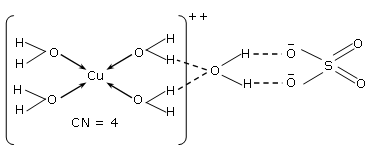Question 20. In a basic medium, H2O2 exhibits which of the following reactions?

A. Mn2+ → Mn4+

B. I2 → I-

C. PbS → PbSO4

1. a. (A), (C) only
2. b. (A) only
3. c. (B) only
4. d. (A), (B) only

Solution: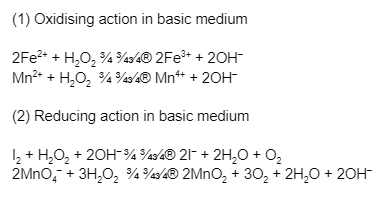SECTION - B

Question 21. The solubility of CdSO4 in water is 8.0 × 10–4 mol L–1. Its solubility in 0.01 M H2SO4 solution is ______ × 10–6 mol L–1. (Round off to the Nearest Integer).

Assume that solubility is much less than 0.01 M)

Solution:

CdSO4(s) ⇌ Cd+2 (aq) + SO42– (aq)

S S

S = 8 × 10–4 Ksp = S2 = 64 × 10–8

CdSO4(s) ⇌ Cd+2 + SO42–

S S + 10–2

Ksp(CdSO4) = 64 × 10–8 = s(s + 10–2)

64 × 10–8 ≃ s × 10–2

s = 64 × 10–6

Question 22. The molar conductivities at infinite dilution of barium chloride, sulphuric acid and hydrochloric acid are 280, 860 and 426 S cm2 mol–1 respectively. The molar conductivity at infinite dilution of barium sulphate is _____ S cm2 mol–1. (Round off to the Nearest Integer).

Solution:

$$\lambda_{M}^{\infty}(BaCl_{2}) = 280$$

$$\lambda_{M}^{\infty}(H_{2}SO_{4}) = 860$$

$$\lambda_{M}^{\infty}(HCl) = 426$$

$$\lambda_{M}^{\infty}(BaSO_{4}) = ??$$

$$=\lambda_{M}^{\infty}(H_{2}SO_{4}) + \lambda_{M}^{\infty}(BaCl_{2}) - 2\times \lambda_{M}^{\infty}(HCl)$$

= 860 + 280 – 2 × 426

= 288

Question 23. A reaction has a half life of 1 min. The time required for 99.9% completion of the reaction is _____ min. (Round off to the nearest integer) [ Use ln2 = 0.69, ln10 = 2.3]

Solution:

t99.9% = 10 x t(1/2) = 10 min

Derivation

$$t_{99.9%} =\frac{1}{K}ln\left (\frac{100}{0.1} \right ) = \frac{1}{K}ln(1000)$$

$$\frac{3}{K}ln (10) = 3\frac{t_{1/2}}{ln(2)}\times ln(10)$$

=

$$3\times (1\: min)\times \frac{ln(10)}{ln(2)}$$

=

$$\frac{3}{log(2)} = \frac{3}{0.3}; 10\: min$$

The gas phase reaction

2A (g) → A2(g)

at 400 K has ΔGº = + 25.2 kJ mol–1

Question 24. The equilibrium constant KC for this reaction is ______ × 10–2. (Round off to the Nearest Integer).

[Use : R = 8.3 J mol–1 K–1, ln 10 = 2.3

log10 2 = 0.30, 1 atm = 1 bar]

[antilog (– 0.3) = 0.501]

Solution:

Using formula

DG° = –RTlnKP

25200 = –2.3 × 8.3 × 400 log (KP)

KP = 10–3.3 = 10–3 × 0.501

= 5.01 × 10–4 Bar–1

= 5.01 × 10—5 × 10–4 Pa–1

=

$$\frac{K_{c}}{8.3\times 400}$$

KC = 1.66 × 10–5m3/mole

= 1.66 × 10–2 L/mol

= 2

Question 25. Consider the below reaction where 6.1 g of benzoic acid is used to get 7.8 g of m-bromo benzoic acid.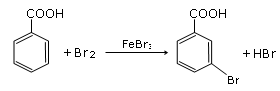The percentage yield of the product is _____

(Round off to the nearest integer)

[Given : Atomic masses : C : 12.0 u, H : 1.0 u, O : 16.0 u, Br : 80.0 u]

Solution: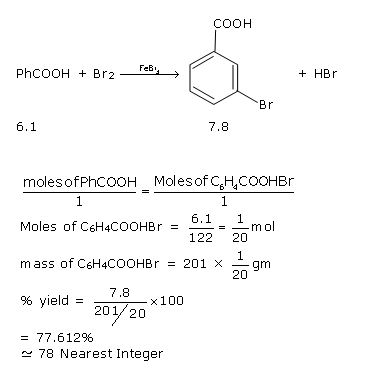Question 26. A solute A dimerizes in water. The boiling point of a 2 molal solution of A is 100.52ºC. The percentage association of A is ______. (Round off to the nearest integer.)

[Use : Kb for water = 0.52 K kg mol–1

Boiling point of water = 100ºC]

Solution:

N = ½

m = 2; Tb soln. = 100.52

ΔTb = 0.52

= i × Kb × m

0.52 = i × 0.52 × 2

i = ½ = 1 + 1 + (½ - 1)α

α/2 = ½

α = 1

Question 27. The number of species below that has two lone pairs of electrons in their central atom is ______. (Round off to the Nearest Integer.)

SF4, BF4, CIF3, AsF3, PCl5, BrF5, XeF4, SF6

Solution:

ClF3 and XeF4 have two lp-in their central atom

Question 28.10.0 mL of Na2CO3 solution is titrated against 0.2 M HCl solution. The following litre values were obtained in 5 readings 4.8 mL, 4.9 mL, 5.0 mL, 5.0 mL and 5.0 mL. Based on these readings and the convention of titrimetric estimation the concentration of Na2CO3 solution is ____mM

Solution:

Na2CO3 + HCl ¾ →

10ml 0.2M

M = ? 5ml

Meq. of Na2CO3 = Meq. of HCl

M × 10 × 2 = 0.2 × 5 × 1

M = 5 × 10-2 M = 50 × 10–3M = 50 mM

Question 29. In Tollen’s test for aldehyde, the overall number of electron(s) transferred to the Tollen’s reagent formula [Ag(NH3)2]+ per aldehyde group to form silver mirror is ________. (Round off to the Nearest Integer)

Solution:

$$R - CHO\overset{2[Ag(NH_{3})_{2}]^{+}OH^{\odot }}{\rightarrow}RCOOH+2Ag+2NH_{3}+H$$

$$2Ag^{+}\overset{2e^{-}}{\rightarrow}2Ag$$

Question 30. A xenon compound ‘A’ upon partial hydrolysis gives XeO2F2. The number of lone pairs of electrons presents in compound A is ______. (Round off to the Nearest Integer).

Solution:

Partial Hydrolysis

XeF6 + H2O → XeOF4 + 2HF

XeF6 + 2H2O → XeO2F2 + 4HF

Complete hydrolysis

XeF6 + 3H2O → XeO3 + 6HF

### JEE Main 2021 Chemistry Paper March 18 Shift 2